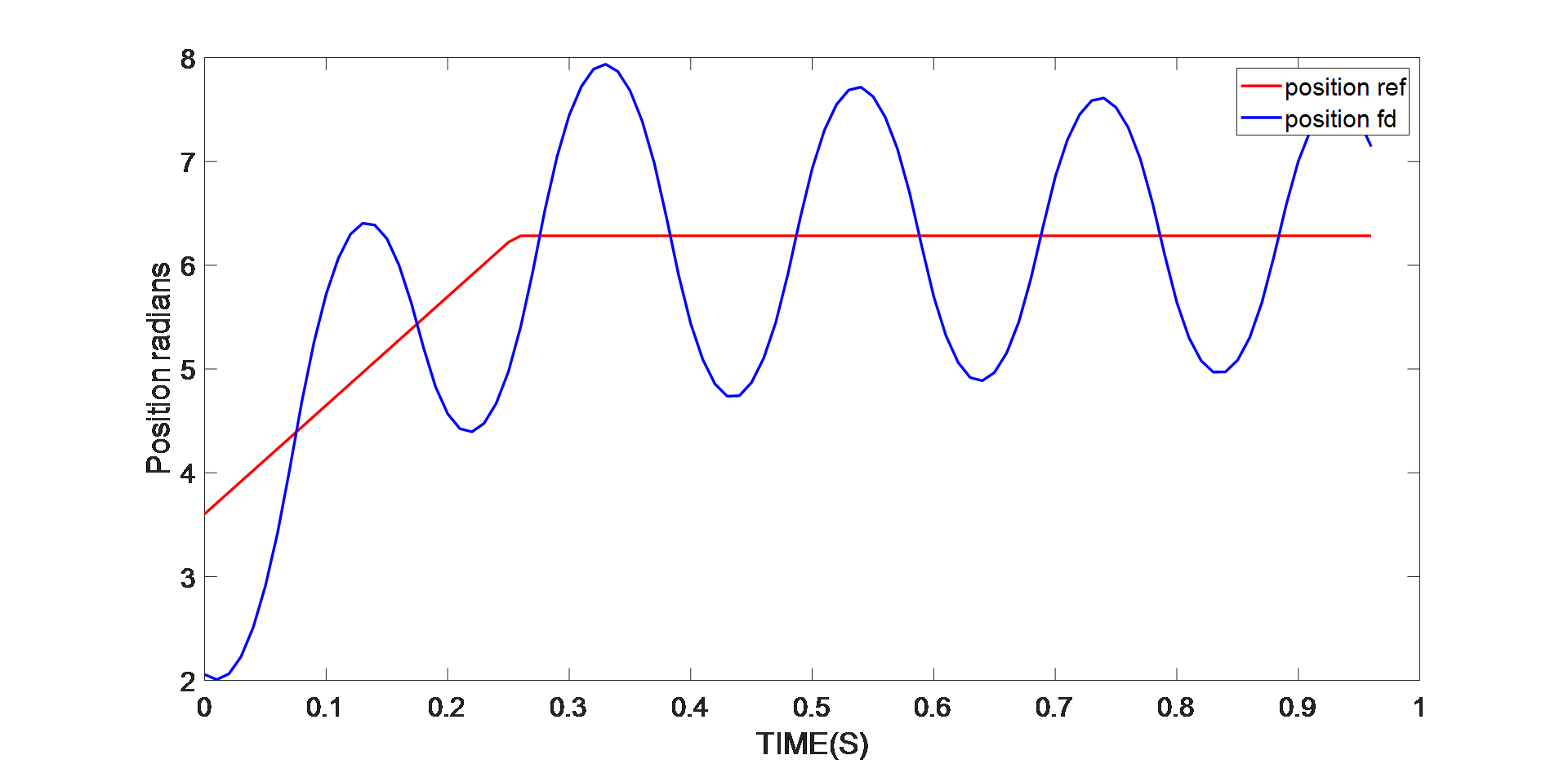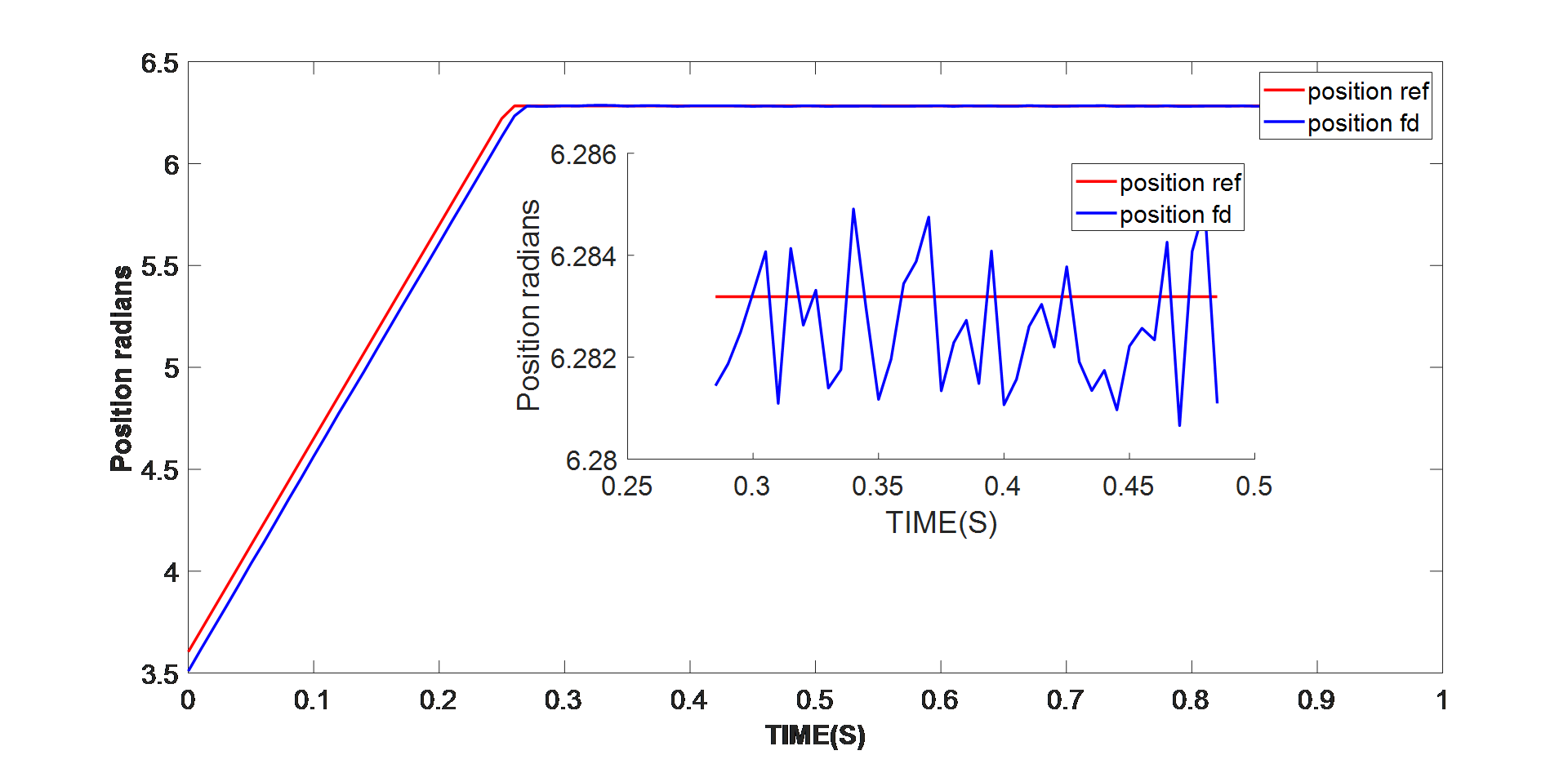# 智能电机的 PMSM 参数识别## PMSM的磁场定向控制（FOC）

FOC 的基本思想是能够分别控制磁通量和扭矩，类似于控制直流电机的方式。根据 Clarke 和 Park 变换，同步旋转 Q-D 坐标系下的 PMSM 模型可以使用等式（1）、等式（2）、等式（3）和等式（4）来计算：

$$v_{QS}=r_S+ω_Rλ_{DS}+ρλ_{QS}$$
$$v_{DS}=r_{S}-ω_rλ_{QS}+ρλ_{DS}$$
$$λ_{QS}=L_Si_{QS}+L_Mi_{QR}$$
$$λ_{DS}=L_Si_{DS}+L_M i_{DR}$$

$$λ_{QS}=L_Si_{QS}$$
$$λ_{DS}=L_Si_{DS}+λ_M^{'}$$

$$T_E= \frac {3} {2} \frac {P}{2} (λ'_{M}i_{QS} + (L_{D} - L_{Q}) i_{DS}i_{QS})$$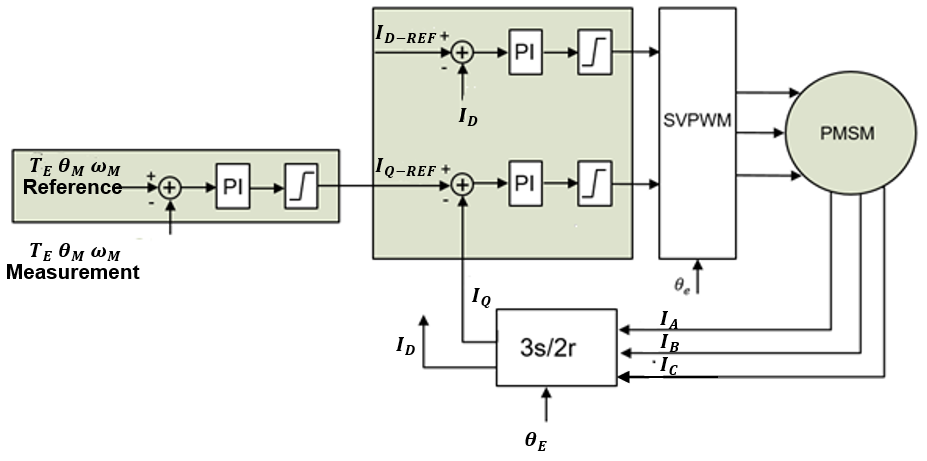D 轴电流参考值 (ID-REF) 根据磁通量要求来设置。电流调节器/控制器的输出（VQ-REF和VD-REF）为空间矢量 PWM (SVPWM) 的输入。SVPWM 模块为逆变器生成栅极信号以驱动 PMSM。

$$G = \frac{KPS+KI} {S} \frac {1}{L_{S}S + r_S}$$

$$G = \frac{KPS+K1} {S} \frac {kt}{JS + B}$$

## 递归最小二乘算法

$$y(t)=ϕ^T (t)θ(t)$$

$$J(θ,t)= \frac {1}{2} ∑_{i=1}^t(y(i)-\phi^T (i) \hatθ (i))$$

P 和 L 的新矩阵可以分别用等式 (12) 和等式 (13) 来计算：

$$P^{-1} (t)=∑_{i=1}^t\phi(i)\phi^T(i)$$ $$L(t)=P(t)\phi(t)$$

$$ϵ(t)=(y(t)-\phi^T (t)) \hatθ (t-1)$$
$$L(t)=P(t-1)\phi(t) (I+\phi^T (t)P(t-1)\phi(t))^{-1}$$
$$P(t)=(I-L(t)\phi^T (t))P(t-1)$$
$$\hatθ(t)=\hatθ (t-1)+L(t)ϵ(t)$$
$$t=t+1$$

$$J(θ,t)=\frac {1}{2} ∑_{i=1}^tλ^{t-i} (y(i)-\phi^T (i)\hat θ (i))$$

$$ϵ(t)=(y(t)-\phi^T (t)) \hatθ (t-1)$$
$$L(t)=P(t-1)\phi(t) (λI+\phi^T (t)P(t-1)\phi(t))^{-1}$$
$$P(t)= \frac {1}{λ}(I-L(t) \phi^T (t))P(t-1)$$
$$\hatθ (t)=\hatθ (t-1)+L(t)ϵ(t)$$
$$t=t+1$$

## 实验结果

MPS’s MMP757188-36 是一款已经过验证的 智能电机。表 1 列出了其数据手册中的电机参数。

 定子相电阻 300mΩ 定子相电感 350µH 电机惯量 410gxcm2 扭矩常数 57mN x m/A 极对数 4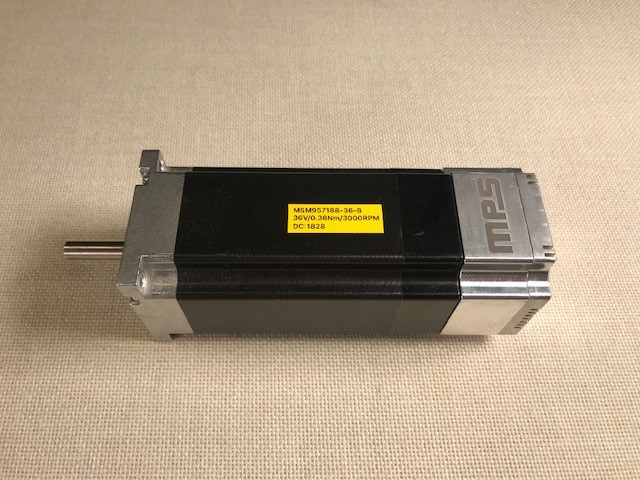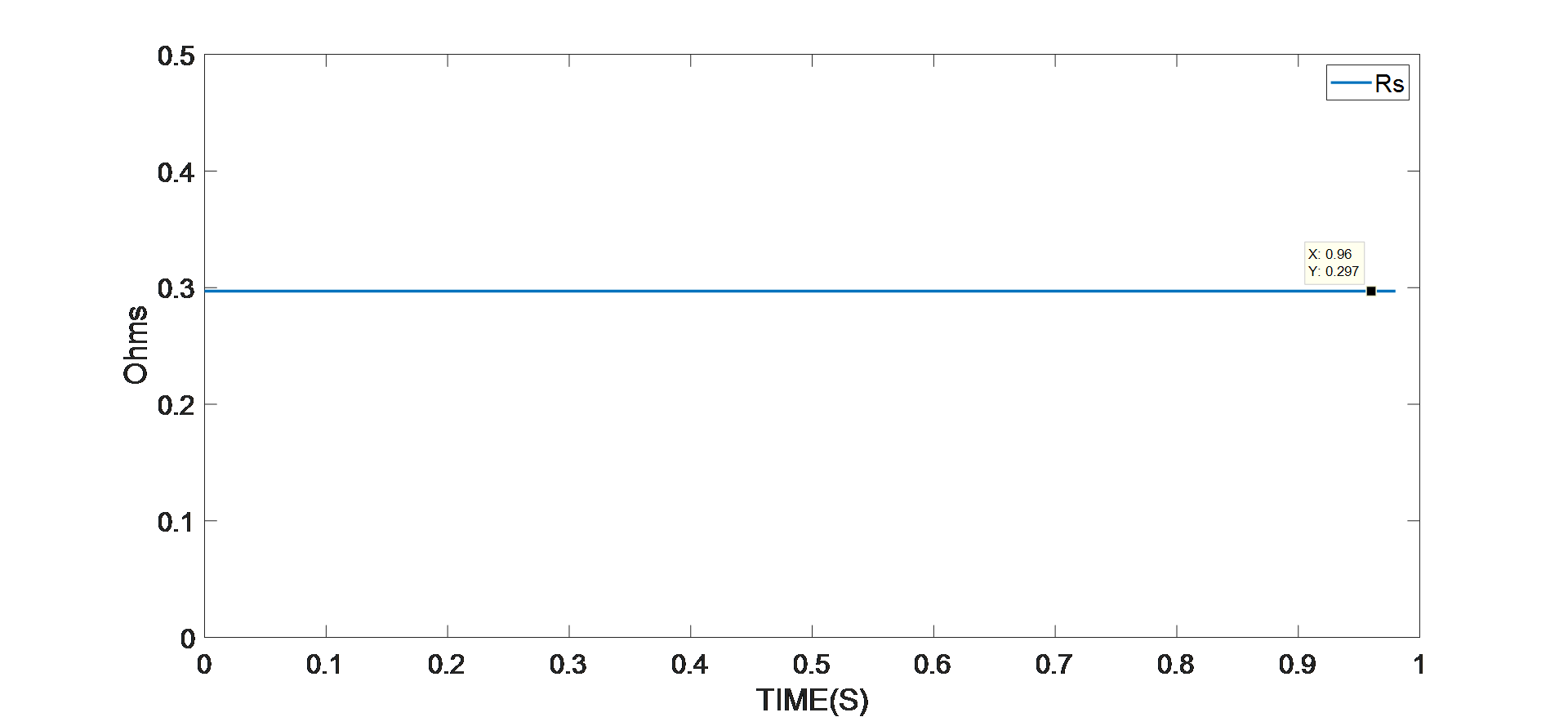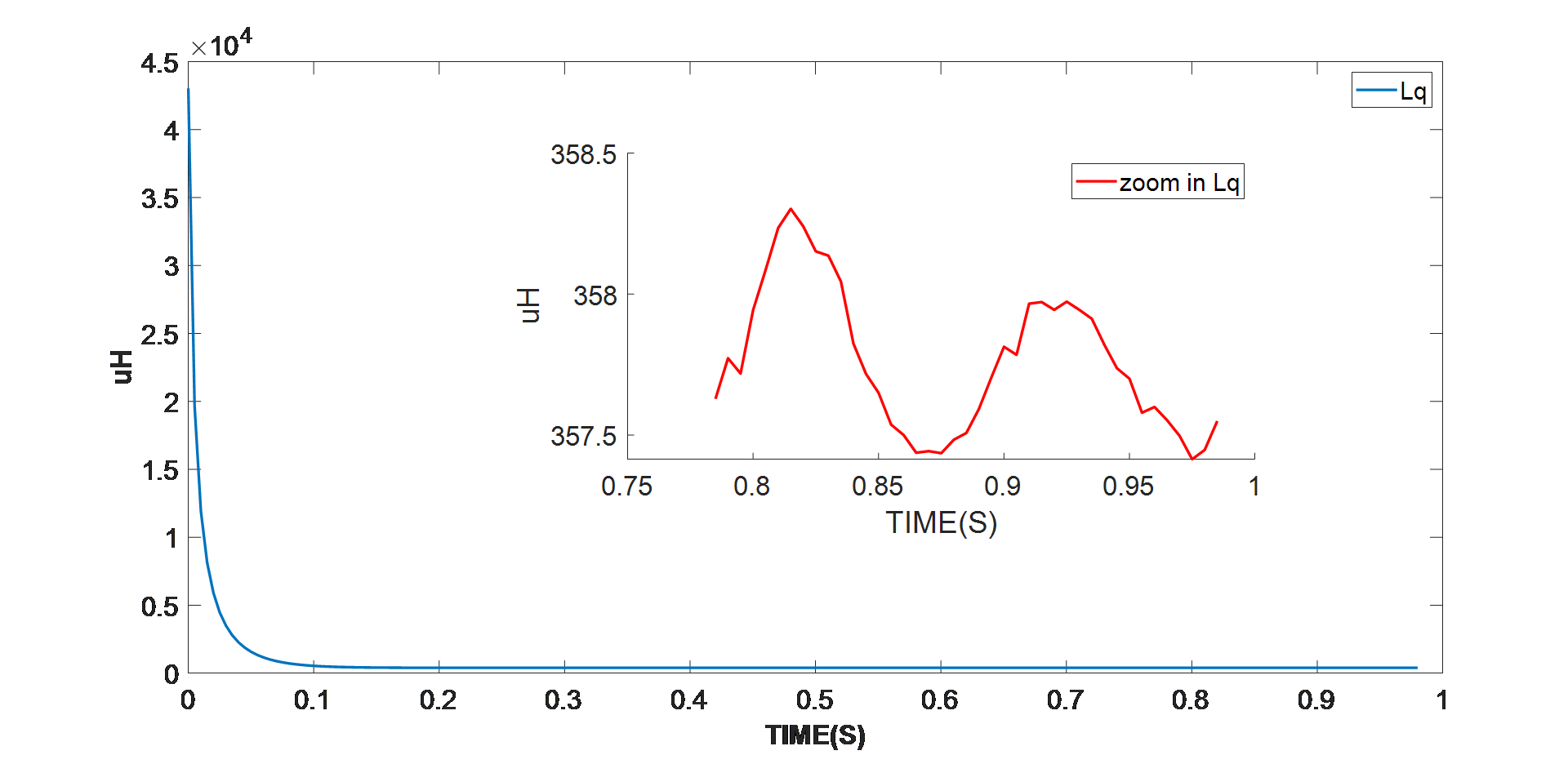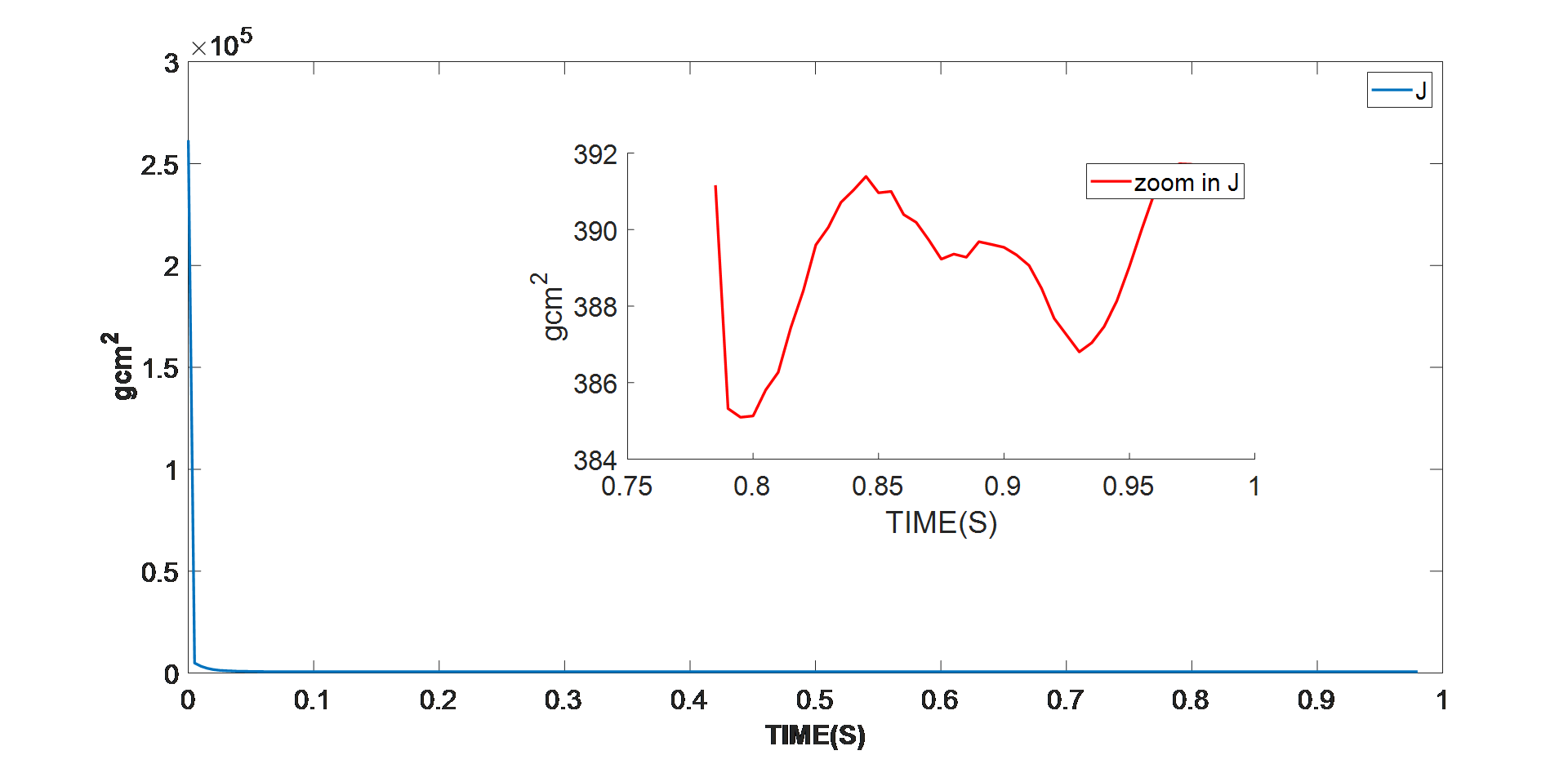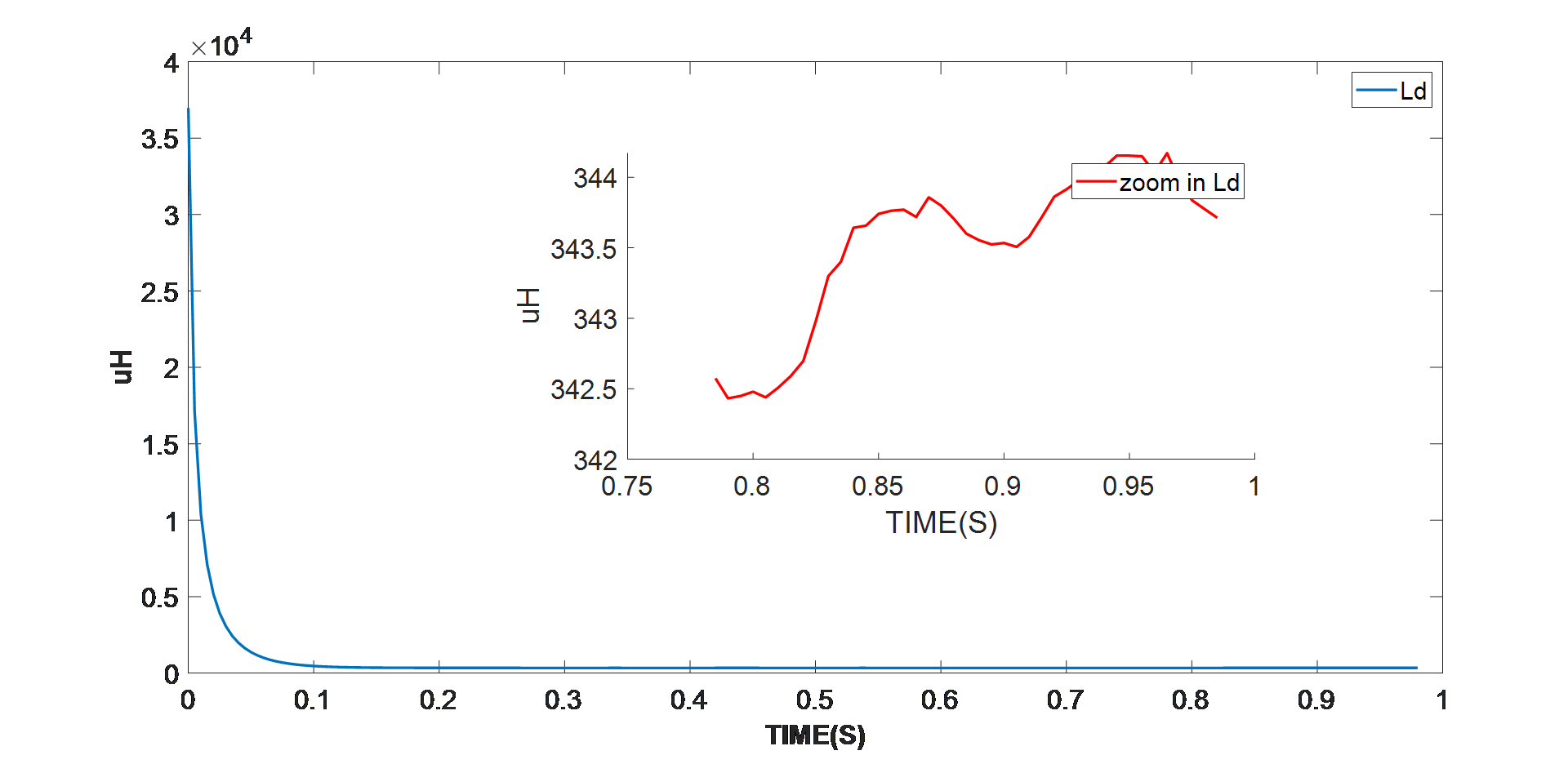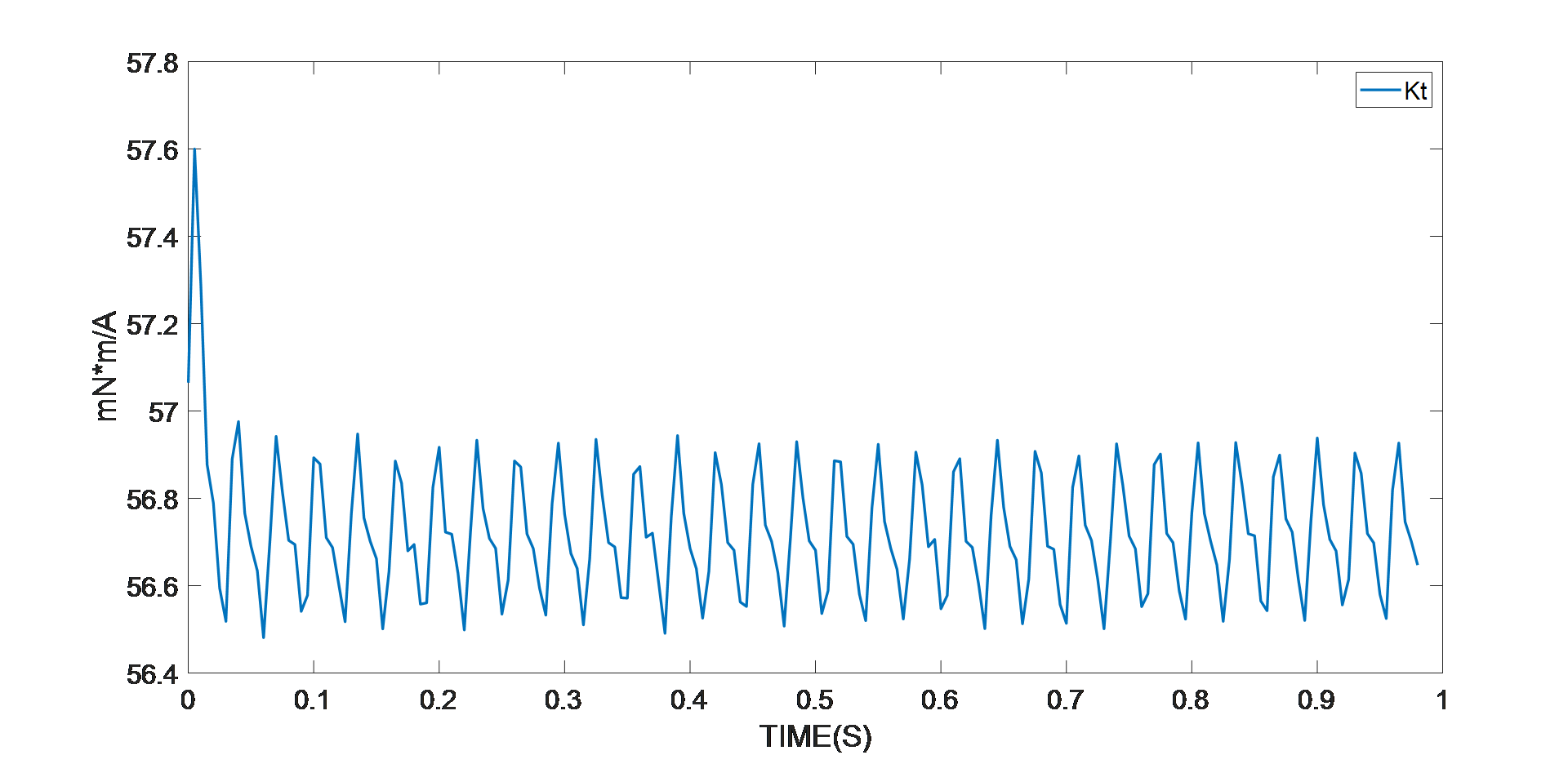定子相电阻 297mΩ D 轴电感 343µH Q 轴电感 358µH 电机惯量 390gxcm2 扭矩常数 57mNxm/A 极对数 4

## 控制环自整定

PMSM 的控制传递函数高度依赖电机参数。如果电机参数不正确，电机将无法有效运行。在下面关于改变轴惯量 (J) 的示例中，我们将进一步讨论这个问题。

PMSM 通常被用作高性能伺服电机。它们的工作条件视情况而异。工程师可能有准确的电机参数数据手册，也有可能需要手动测量电机参数。一旦将电机置于复杂的机械系统中，就很难再确定轴惯量。

MPS’s MMP757188-36 可用于驱动旋转盘（见图 8）。旋转盘的轴惯量从410 gxcm2增加到 7100 gxcm2。FOC设计为位置带宽是20Hz，速度带宽是200Hz，电流带宽是2000Hz。